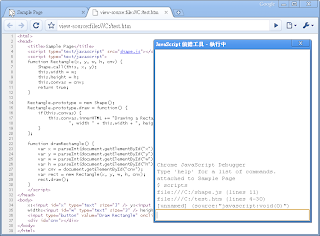### 在 Chrome 2.0 中為 JavaScript 偵錯

Chrome 不僅滿足使用者對瀏覽器的根本訴求，也從開發人員需求實務上的觀點設計許多的功能，希望能藉以協助開發人員提供更好的使用者經驗。在開發人員的工具中，最令我感興趣的，卻也是對我極度不友善而讓我望之卻步的，莫過於基於命令提示介面的 JavaScript 偵錯工具（JavaScript Debugger）了。當然，這對於既自詡為 Chrome 愛用者，又身兼網頁開發人員的我來說，似乎令人難以悅服。於是，最近我又再度興起要深入研究這個好用的小工具的念頭，如今在此分享自己的學習心得及蒐集的相關資訊，希望能對有興趣的朋友有所幫助。

b[reak] <function | script:function | script:line | script:line:pos> [condition]在來源中的位置或函式設定中斷點。
break_info [breakpoint #]

bi [breakpoint #]

clear <breakpoint #>移除已指定的中斷點。
h[elp] [command]顯示所有命令的描述，或是已指定命令的詳細描述。
p[rint] <expression>評估運算式並輸出執行結果。
scripts顯示所有可偵錯的指令碼資訊。

args顯示目前函數的參數。
b[reak] [<function | script:function | script:line | script:line:pos> [condition]]在來源中的位置或函式設定中斷點，或是不使用任何參數停止偵錯並終止程式執行。
break_info [breakpoint #]

bi [breakpoint #]

backtrace [from frame #] [to frame #]

bt [from frame #] [to frame #]

clear <breakpoint #>移除已指定的中斷點。
c[ontinue]繼續執行到下一個中斷點。
dir <expression>顯示物件的詳細資訊。
f[rame] <frame #>顯示目前堆疊框架（Stack Frame）的偵錯資訊，或是已指定堆疊框架的偵錯資訊。
h[elp] [command]

? [command]

locals顯示目前堆疊框架中所有變數的值。
n[ext]逐步執行每行指令碼，如果是執行函式呼叫，則會進入函式內的第一行指令碼。
p[rint] <expression>評估運算式並輸出執行結果。
scripts顯示所有可偵錯的指令碼資訊。
source [from line] | [<from line> <num lines>]

ls [from line] | [<from line> <num lines>]

s[tep]逐步執行每行指令碼，如果是執行函式呼叫，則不會進入函式直接執行。
stepout

so

`<html><head>    <title>Sample Page</title>    <script type="text/javascript" src="shape.js"></script>    <script type="text/javascript">         function Rectangle(x, y, w, h, cnv) {        Shape.call(this, x, y);        this.width = w;        this.height = h;        this.convas = cnv;        return true;    }            Rectangle.prototype = new Shape();    Rectangle.prototype.draw = function() {        if(this.convas) {            this.convas.innerHTML += "Drawing a Rectangle at:" + this.getCoordinates() +                    ", width " + this.width + ", height " + this.height + "<br/>";        }    };        function drawRectangle() {        var x = parseInt(document.getElementById("x").value);        var y = parseInt(document.getElementById("y").value);        var w = parseInt(document.getElementById("w").value);        var h = parseInt(document.getElementById("h").value);        var cnv = document.getElementById("cnv");        var rect = new Rectangle(x, y, w, h, cnv);        rect.draw();    }    </script></head><body>    x:<input id="x" type="text" size="3" /> y:<input id="y" type="text" size="3" />    width:<input id="w" type="text" size="3" /> height:<input id="h" type="text" size="3" />    <input type="button" value="Draw Rectangle" onclick="javascript:drawRectangle();" />    <div id="cnv"></div></body></html>`

`function Shape(x, y) {    this.x = x;    this.y = y;    return true;}Shape.prototype = {    getCoordinates : function() {        return "(" + this.x + " ," + this.y + ")";    }};``\$ scriptsfile:///C:/shape.js (lines 11)file:///C:/test.htm (lines 4-30)[unnamed] (source:"javascript:void(0)")`

`\$ break Shapeset breakpoint #1`

`var rect = new Rectangle(x, y, w, h, cnv);`

`\$ break file:///C:/test.htm:28set breakpoint #2`

`\$ break file:///C:/test.htm:24 x<0set breakpoint #3`

`var y = parseInt(document.getElementById("y").value);`

`\$ break_infoNum breakpoints: 3id=1, hit_count=0, type=function, target=Shapeid=2, hit_count=0, type=script, target=file:///C:/test.htm, line=27id=3, hit_count=0, type=script, target=file:///C:/test.htm, line=23, condition=x<0`

`paused at breakpoint 3: drawRectangle(), file:///C:/test.htm24:         var y = parseInt(document.getElementById("y").value);`

`\$ next25:         var w = parseInt(document.getElementById("w").value);`

`\$ continuepaused at breakpoint 2: drawRectangle(), file:///C:/test.htm28:         var rect = new Rectangle(x, y, w, h, cnv);`

`\$ localsw = 400cnv = #<an HTMLDivElement>x = -10y = 0h = 600rect = undefined`

`\$ continuepaused at breakpoint 1: #.Shape(x=-10, y=0), file:///C:/shape.js2:     this.x = x;`

`\$ backtraceFrames #0 to #4 of 5:#00 #<an Object>.Shape(x=-10, y=0) file:///C:/shape.js line 2 column 12 (position 36)#01 new Rectangle(x=-10, y=0, w=400, h=300, cnv=#<an HTMLDivElement>) file:///C:/test.htm line 7 column 15 (position 58)#02 drawRectangle() file:///C:/test.htm line 28 column 20 (position 839)#03 #<an HTMLInputElement>.[anonymous](evt=#<a MouseEvent>) file:///C:/test.htm line 37 column 12 (position 177)#04 #<an HTMLInputElement>.onclick(evt=#<a MouseEvent>) file:///C:/test.htm line 38 column 4 (position 197)`

`\$ frame 1#01 Rectangle, undefined7:         Shape.call(this, x, y);`

`\$ source 5:  6:     function Rectangle(x, y, w, h, cnv) {>>>>        Shape.call(this, x, y); 8:         this.width = w; 9:         this.height = h;10:         this.convas = cnv;11:         return true;12:     }`

`\$ breakJavaScript execution already stopped.`

Sample debug session with Google Chrome JavaScript debuger
Basic information on Chrome's Debugger